PHYSICS

Mass

MASS

MA. Fundamental of Mass MB. Kong Frequency and Kong Wavelength MC. Annihilation and Pair Production

MD. Kong Equation

ME. Kong Atom Model MF. Quantum of Atom MG. Perturbation of Photon

MH. Periodic Table

MI. Chemical Reaction MJ. Superconductor MK. Particles and Waves ML. Nuclear Physics

MF. QUANTUM OF ATOM

1. Principle Orbital Number 2. Sub-Orbital Number 3. Number of Electrons
5. Addresses of Electrons 6. Relation of Schrödinger Equation

INTRODUCTION

In this chapter, we discuss about the addresses of the atom. The Kong Equation plays an important role in solving the quantum mechanics of atom. It gives solutions to the 3 quantum numbers of atom. The 3 quantum numbers are the:-

1) Principle Orbital Number (PON),

2) Sub-Orbital Number (SON), and

3) Electron Number (EN).

These 3 quantum numbers are used to address and identify the electron in an atom; the symbols that allocated for them are,

1) Principle Orbit Number, n

2) Sub-Orbital Number, l, and

3) Electron Number, Ne

OBJECTIVES

1)      To develop an addressing system for the electrons in an atom

2)      To study the relation between the Kong equation and the Schrödinger equation.

MF.1.0        PRINCIPLE ORBITAL NUMBER, PON

The first quantum number is the principle orbital number, which is given by the symbol n. The value is from 1, 2, 3, …, ∞. PON is the major orbital for the energy transition. For electrons travel from one PON to another PON, photon energy is released or absorbed.

From the chapter “Kong Equation”, the PON, n, is introduced; we quote from eq. MD.3.21 as follow,

ra = n rB                                                                                               … eq. MF.1.1

n = principle orbital number = 1, 2, 3, …, ∞

From the Kong atom model, it is also developed that the principle energy level depends on the PON. For hydrogen atom, the energy level at each PON is,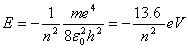n = 1, 2, 3… ¥                                  … eq. MF.1.2

MF.2.0        SUB-ORBITAL NUMBER, SON

The second quantum number is the sub-orbital number, which is given by the symbol l. It carries the value of l £ n or l = 1, 2, 3… n.

From the solution of the Time Independent Kong equation, table MD.3.1 and the Kong atom model, we know that at higher PON, the allowable tilting angle is higher. This implies that the area of the magnetic Gaussian surface is higher and can allow for more SON. Larger magnetic Gaussian surface and more numbers of sub-orbital can house more electrons in the atom.

For n = 1, only one sub-orbital is allowed, which is l = 1 at angle 90°. For n ³ 2, 2 additional sub-orbitals are allowed by each increment of PON. The total number of sub-orbitals for a given principle orbit number can be expressed as follow,

ln = 2n – 1    n = 1, 2, 3… ¥                                                                … eq. MF.2.1

For multi-electrons atom, at higher PON, n, the atom can house for more electrons. All the electrons in the atom are negatively charged. The planar electric force is balanced by the magnetic force, but the vertical electric repulsive force between electrons causes the orbit to tilt vertically at higher angle to achieve stability. The tilted electrons refer to the planar-orbital of the electrons is not perpendicular to the angular momentum or the magnetic dipole moment of the atom. The tilted electrons have higher energy level compare to the non-tilted electrons. The higher the tilting angle, the higher the energy level. The electrons tend to stay at lower energy and tend to fill the lower energy sub-orbital for better stability.

MF.2.1        Spectroscopic Notation

The orbital quantum number sets the selection rules of electronic transitions. The notation is as follows:

 SON \ PON n = 1 n = 2 n = 3 n = 4 s -- sharp l = 1 1s 2s 3s 4s p -- principal l = 2 2p 3p 4p d -- diffuse l = 3 3d 4d f  -- fundamental l = 4 4f g l = 5 Beyond this point, the notation just follows the alphabet. h l = 6

Table MF.2.1

The electrons tend to fill up from left to right for PON and top to bottom for SON for better stability. However, for higher PON, this is not always the case because the lower SON for the next PON might has lower energy level than the higher SON of the current PON. A comprehensive electrons allocation for the common atoms is postulated and presented in the chapter "Periodic Table".

MF.3.0        NUMBER OF ELECTRONS

From the solution of the Kong equation, it is described that each sub-orbital can house for two electrons. The total number of electrons is given the symbol Ne. The total EN is depending on the number of SON, which gives the value,

Ne = 2ln = 2(2n – 1)        n = 1, 2, 3… ¥                                             … eq. MF.3.1

The total Ne is equivalent to the proton number of atom. The number of electron in each SON is either 1 or 2. Ne = 1 is half filled and Ne = 2 is fully filled.

MF.4.0        ALL THE ATOM QUANTUM NUMBER

All the atom quantum numbers that developed using the Kong equation and the Kong atom model are summarized in table MF.4.1 below. The first 7 PON is presented.

 PON, n Allowable tilting angle, q SON, l £ n Total Number of Sub-Orbitals, ln = 2n – 1 Total Number of Electrons, Ne = 2ln 1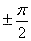1 1 2 2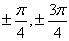1, 2 3 6 3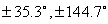1, 2, 3 5 10 41, 2, 3, 4 7 14 5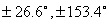1, 2, 3, 4, 5 9 18 6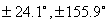1, 2, 3, 4, 5, 6 11 22 7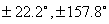1, 2, 3, 4, 5, 6, 7 13 26

Table MF.4.1

By knowing the 3 quantum numbers, the address of an electron in atom can be determined. Figure MF.5.1 suggest the three-dimensional orbital of an atom for the first 3 PON. The higher the PON, the higher the tilting angle and the more the SON.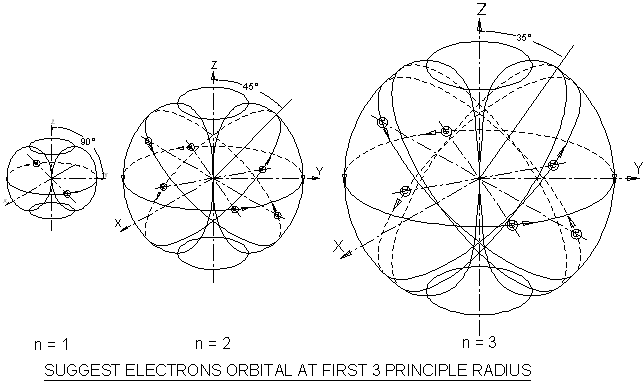Figure MF.5.1

Figure MF.5.2 is a vector model showing the allowable tilting angle of SON. In certain cases, the electrons may appear on the higher PON because the lower SON for the next PON might has lower energy level than the higher SON of the current PON. For multi-electrons atom, the electrons may not fully fill all the SON at lower PON such as transition metal. More discussions are described in the chapter “Periodic Table”.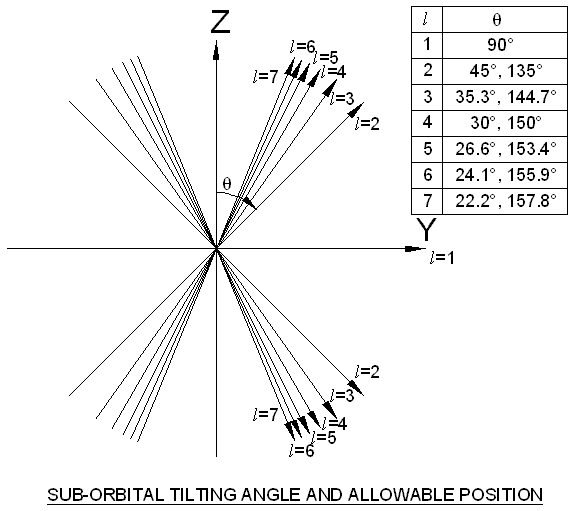Figure MF.5.2

Electrons located at l = 1 has good stability because of no repulsive force between the electrons or the repulsive forces between electrons are balanced. In addition, the electrons orbit at l = 1 are perpendicular to the direction of the magnetic dipole moment of atom. In this phenomenon, the circulating electron generates the highest magnetic moment, me, in order to 'neutralize' the magnetic moment of the atom. The resultant magnetic moment of the atom is reduced and this increases the magnetic stability of the atom.

An example is shown for the atom Lithium. Lithium has the proton number of 3 and has 3 electrons; the address of the electrons is tabulated in table MF.5.1 below,

 3 Li Description n = 2, 1 Lithium has PON = 2 and 1 l = 1, 1 Electrons orbit at angle 90º for both PON Ne = 1, 2 Orbit PON=1 and SON=1 is fully filled with two electrons, the third electron fill at PON=2 and SON=1

Table MF.5.1

MF.6.0        RELATION OF SCHRÖDINGER EQUATION

Presently, the “solutions” for Schrödinger equation is used to describe the quantum mechanics of atom. Here, we relate them with the quantum mechanics of atom from the solutions of the Kong equation and the Kong atom model.

In the solutions of Schrödinger equation base on the wave model, the assignments of the solutions to the value of quantum mechanics are arbitrary. The quantum mechanics developed through Schrödinger equation are:-

1)      The principle quantum number, n,

2)      The orbital, l,

3)      The angular momentum L,

4)      The magnetic quantum number, ml.

From the solution of Schrödinger equation, the assignment of the magnitude of the orbital angular momentum is given as follow,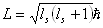, ls = 0, 1, 2…, (n – 1)                                     … eq. MF.6.1

where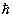= h / 2p = reduced Plank’s constant

The direction of the orbital angular momentum is given as follow,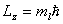, ml = 0, ±1, ±2…, ± l                                     … eq. MF.6.2

The minimum angle occurs when ml = l, where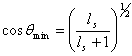, ls = n – 1                                                        … eq. MF.6.3

The first quantum number, the PON was described in chapter MF.1.0 which is similar for both solutions; therefore it will not be discussed again below.

MF.6.1        Vector Angle

Consider an example for the hydrogen atom as described in figure MF.6.1. The electron travels at the PON = n. Figure MF.6.1 shows the projection of the orbital angular momentum, L, using the Kong atom model and the Kong equation.

For the useful vector model described by the Schrödinger equation, the angle is between the orbital angular momentum to the z-axis. But in the Kong atom model explanation and derivations, the angle is between the orbital angular momentum to the y-axis as shown in figure MF.6.1 below.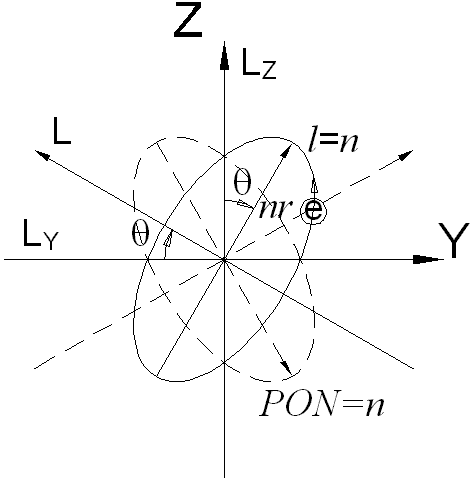Figure MF.6.1

From the solution of the Kong Equation, at PON = n and the highest possible SON, l = n, the tilting angle is expressed as follow,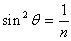… eq. MF.6.4

Trigonometry identity proves that,

sin2q + cos2q = 1                                                                                … eq. MF.6.5

Substitute eq. MF.6.5 into eq. MF.6.4 and rearrange, we obtain,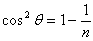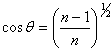… eq. MF.6.6

From eq. MF.6.3, we know that at the highest angular momentum, the orbital from the Schrödinger equation is,

ls = n – 1                                                                                              … eq. MF.6.7

Substitute eq. MF.6.7 into eq. MF.6.6, we obtain,, ls = n – 1                                                        … eq. MF.6.8

Eq. MF.6.8 is equivalent to eq. MF.6.3. It is proven that the Kong equation also produces the same tilting angle, which tally with the Schrödinger equation.

MF.6.2        Orbital, ls

From the Kong atom model, quoted from eq. ME.5.8, the orbital angular momentum for the hydrogen atom has the value of,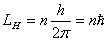… eq. MF.6.9

Referring to figure MF.6.1, the electron circulates at n radius has the orbital angular momentum as described by eq. MF.6.9. We then project the orbital angular momentum to the y-axis, we obtain,

Ly = LH cos q                                                                                       … eq. MF.6.10

Substitute eq. MF.6.6 and MF.6.9 into eq. MF.6.10, we obtain,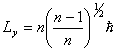… eq. MF.6.11

Rearrange eq. MF.6.11, we obtain,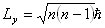… eq. MF.6.12

Substitute eq. MF.6.7 into eq. MF.6.12 and rearrange, we obtain,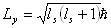, ls = 0, 1, 2…, (n – 1)                                     … eq. MF.6.13

Eq. MF.6.13 is equivalent to eq. MF.6.1 of the solutions of the Schrödinger equation, which once again shows that the proper derivations and the solutions of the Kong equation is valid.

MF.6.3        Direction of Orbital Angular Momentum

For PON higher than 1, the solution of Kong equation produces more than one SON. For SON higher than 1, there are two SONs at equal opposite angle as shown by the solid and dashed line of figure MF.6.1. The projection of orbital angular momentum to the y-axis produces two opposite direction. The two directions are differentiated by positive and negative sign.

For SON = 1, the projection to y-axis is zero. For SON = 2, there are two y-axis projection at two different direction, the direction can be represented with ±1. Similarly for higher SON, the projections can be represented by ± ml.

Therefore, the direction of orbital angular momentum can then be summarized as follow,

Dy = ml                        , ml = 0, ±1, ±2…, ± (n – 1)                            … eq. MF.6.14

where   Dy = direction of orbital angular momentum

Eq. MF.6.14 is equivalent to eq. MF.6.2 for the direction of orbital angular momentum. The direction of the orbital angular momentum of Schrödinger equation only shows the direction of the electrons. In which similarly, the solution of the Kong equation also shows clearly the positive and negative tilting angle as indicated in table MF.4.1.

The proper derivations and the solutions of the Kong equation are equally good in determining the quantum mechanics of atom.

DISCUSSIONS AND CONCLUSIONS

The proper derivations the Kong equation give solutions to the 3 important quantum number of atom. The 3 quantum of atom are the Principle Orbital Number, Sub-Orbital Number and the Electron Number. These 3 quantum numbers are used to address all the electrons in the Kong atom model.

The 3 quantum numbers assigned by the Schrödinger equation can be related to the quantum numbers that derived from the Kong equation and the Kong atom model. In which the Kong equation is equally good in determining the quantum mechanics of atom.

The addressing system of the electrons using the Kong atom model shows good explanations on the characteristic of the atom physically and chemically. This will be discussed in more detail in the chapter "Periodic Table".

This website is originated on 15-Mar-2007,

updated on 4-Jan-2009.